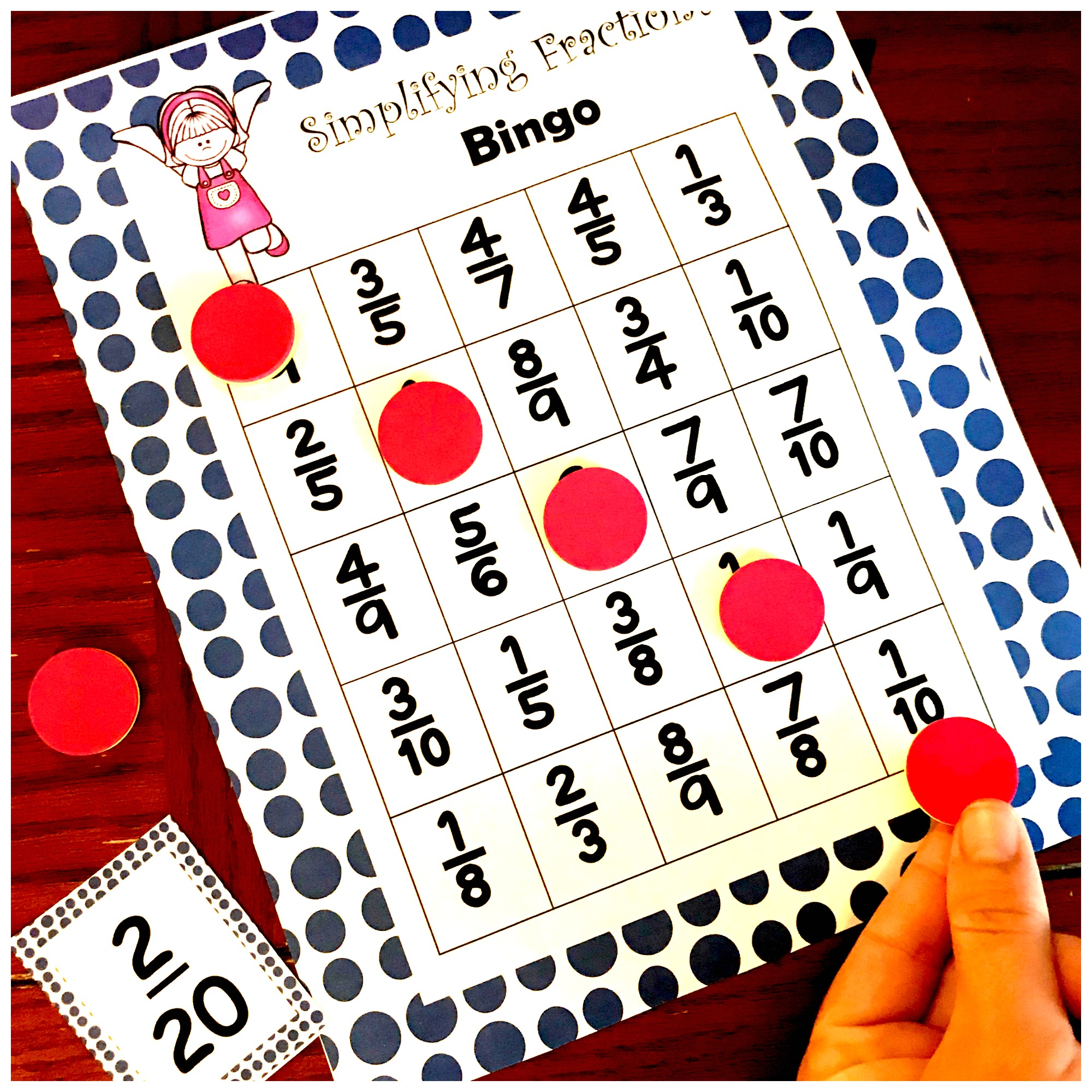Home » Math » Free Printable Fraction Game For Multiplying Fractions

Free Printable Fraction Game For Multiplying Fractions

This FREE printable fraction game is a great way to practice solving fraction by fraction multiplication problems. There are simple expressions to solve, and there are some problems that require students to solve using an area model.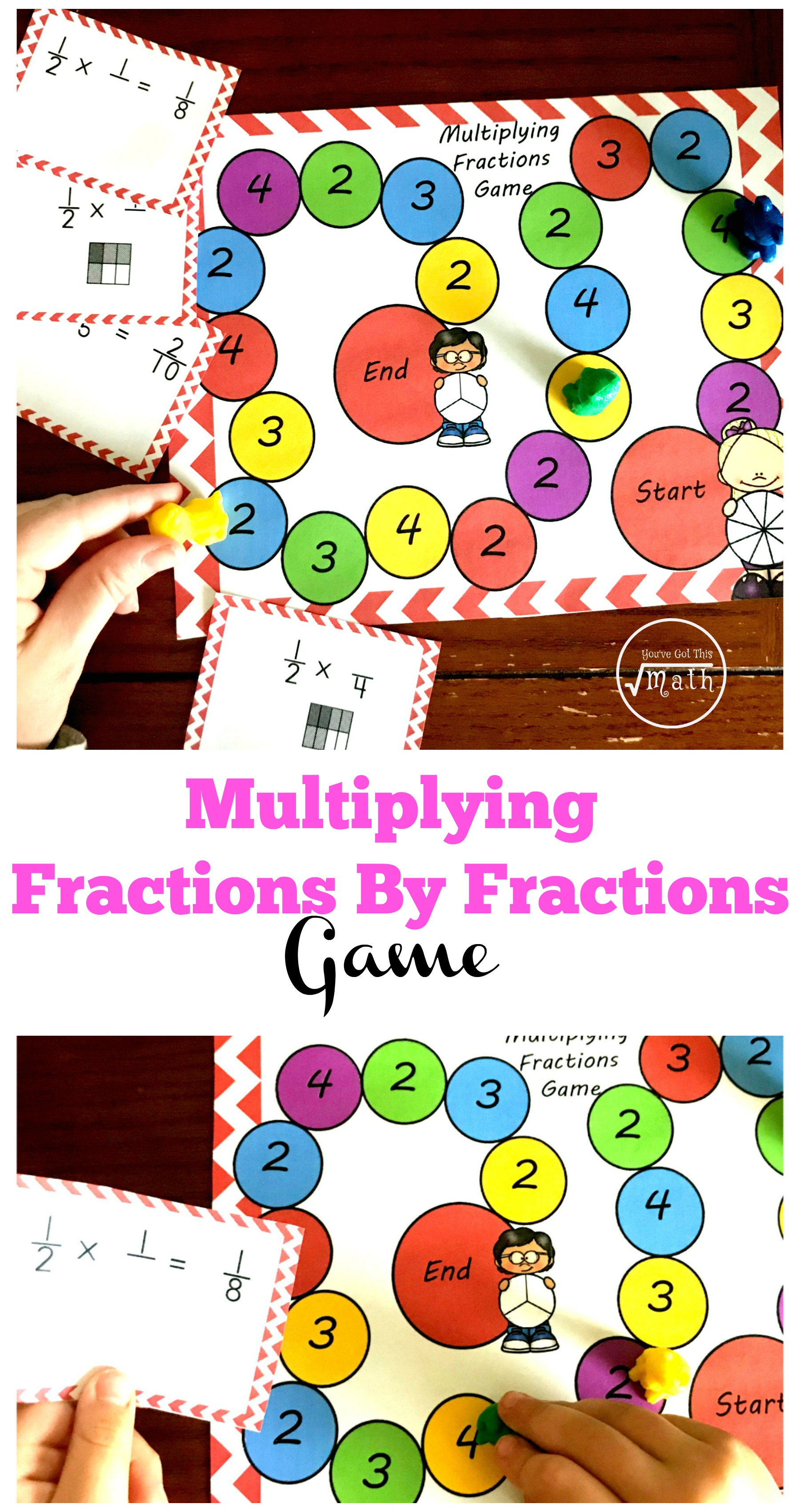There is one thing we as teachers know. Some skills require a lot of practice.

We could pull out a worksheet, but most children don’t enjoy that! And though sometimes you have to have a grade…so a worksheet is needed….practicing math skills on them may not always be the best! That is where games come in.

Human beings need social interaction. That’s why there are book clubs, reading circles, and guided reading groups. These activities make reading social and more fun.

We can do the same with math by allowing children to practice the math skills with their friends. Even children that normally don’t enjoy math may like playing a game with their friends, siblings, teachers, or a parent. Now the practice is fun, and not just a chore.

With all that said, here is a multiplying fraction game.

This is the fourth activity in my multiplying fractions by fractions series. Here are a few of the other activities.

Interactive notebook

BINGO game

Cut and Paste Practice

You can save time and get them in one download!

or get other free printable fraction games HERE.Prep Work –

• First, print off game sheet on card stock paper
• Next, print off game cards on card stock paper, cut out, and laminate.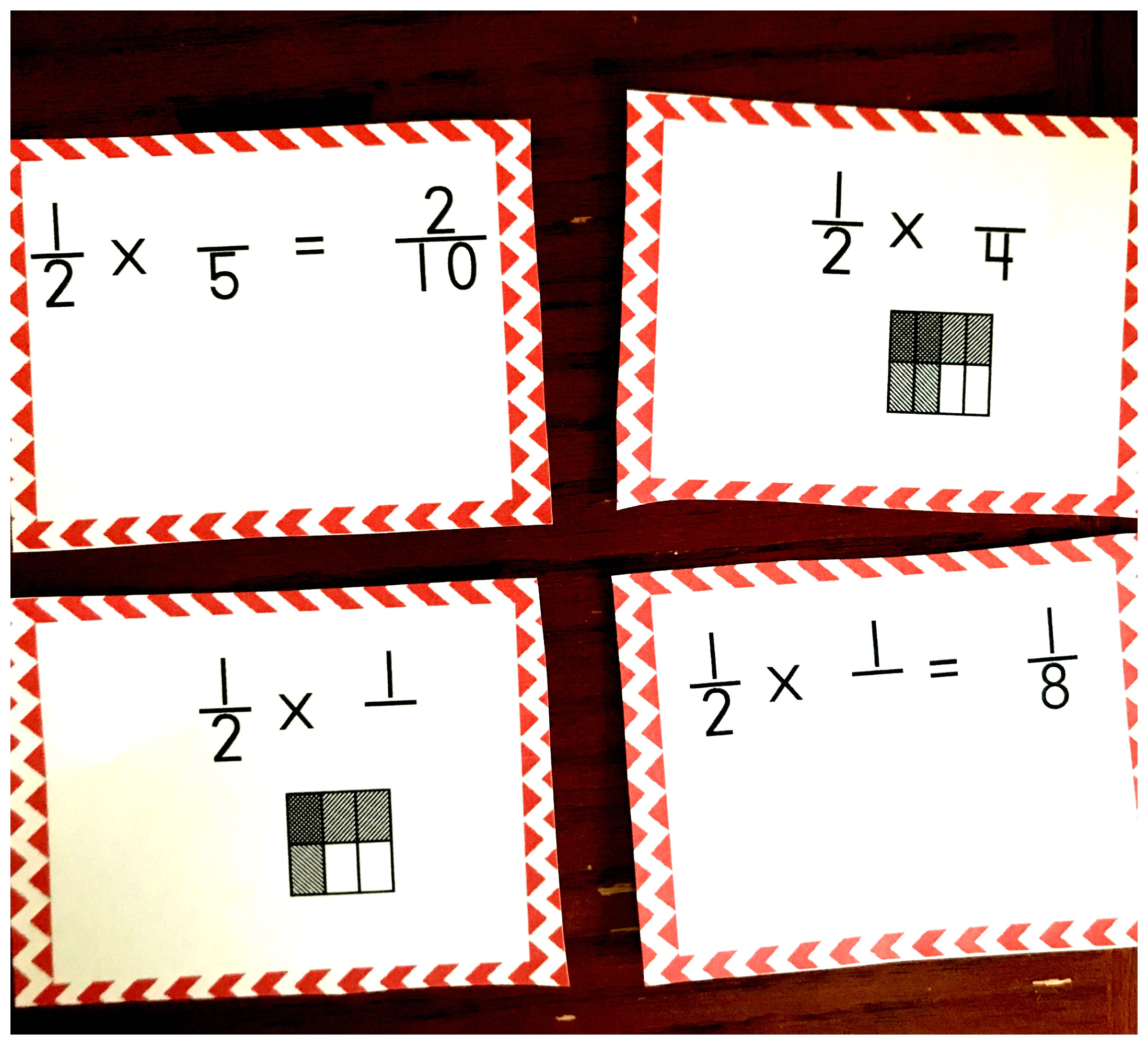• Finally, gather up game pieces and you are ready to go!

Directions –

The directions are very simple!!

First, player one draws a card and figures out the missing numerator or denominator.

In this example, the denominator is missing. The children must look at the first fraction and the answer to figure out the problem. Then they could solve by figuring out what 8 divided by 2 is. Or by asking, “Two times what equals eight?” Our little ones should come up with the answer 4!!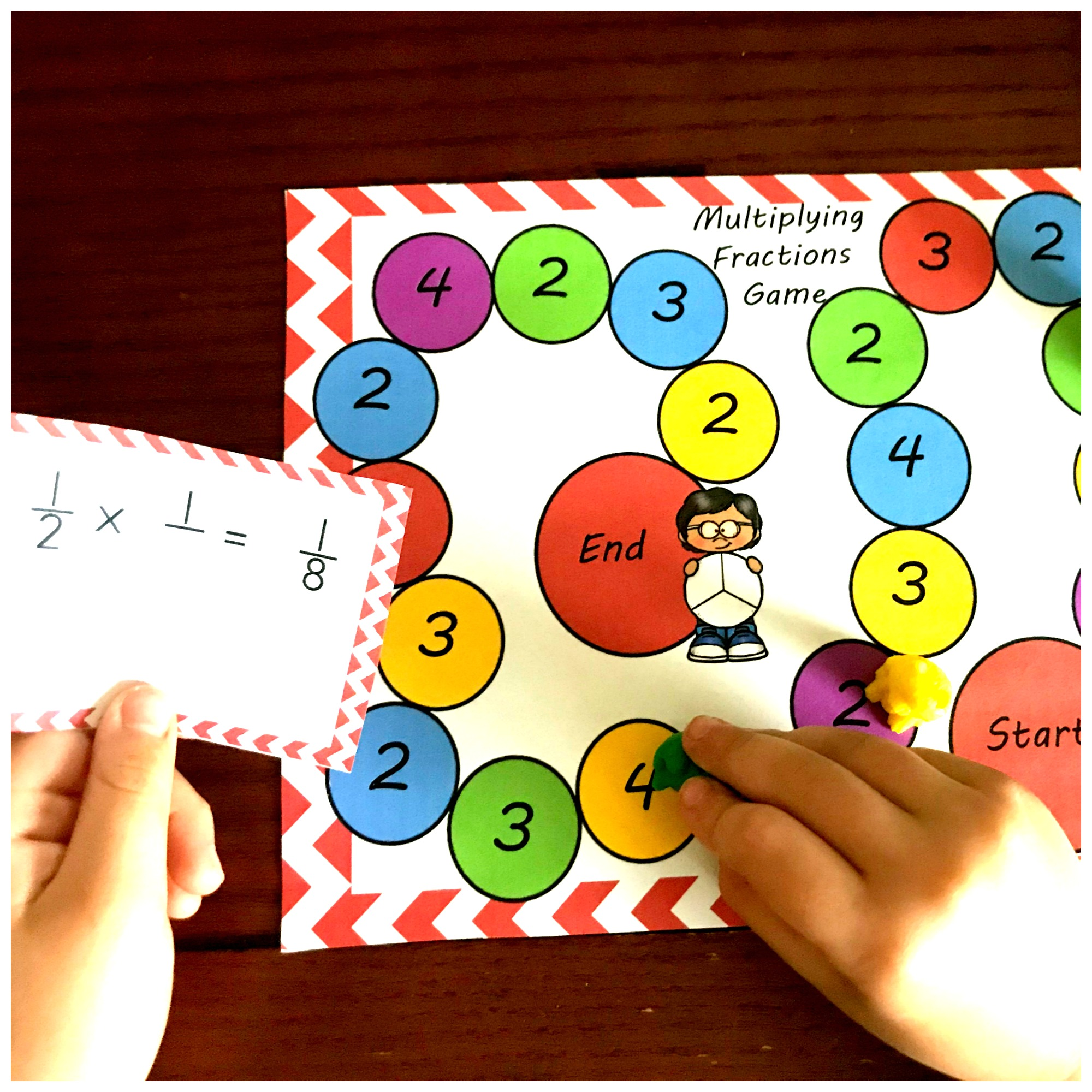Next, the children find the answer to the problem on the game board and move to the first one they come to. In our example, the answer was four, so the player moves to the first four they come to.

Finally, the game ends when they have an answer that is no longer in front of them. That player may then move to the END space, and they are the winner.

Here’s another example!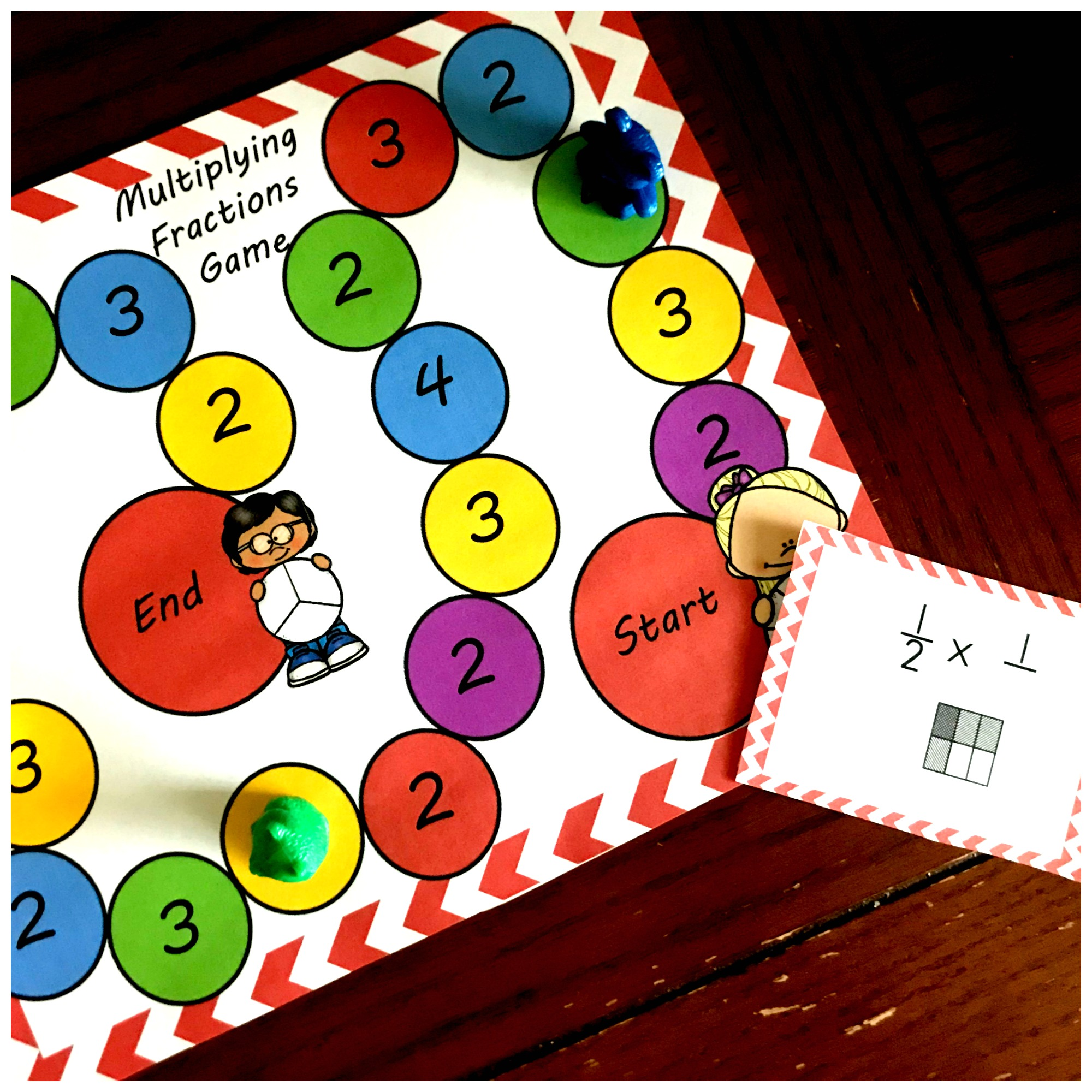These game pieces require knowledge of area models and an understanding of numerators and denominators. In this problem, the denominator is once again missing. The difference is that there isn’t an answer, and they must use the area model to figure out the answer.

If we look at the horizontal lines, we can see that the rectangle has been divided up into 1/2. The first fraction of the expression.

Next, we look at the vertical lines. Now we can see that the rectangle has been divided into thirds, with one of those thirds colored in. Since we are looking for the denominator the answer is 3.

I hope you and your children enjoy this run multiplying fraction game.

You’ve Got This

Rachel

Need other fraction games? You may like….

Multiplying fractions by a Whole Number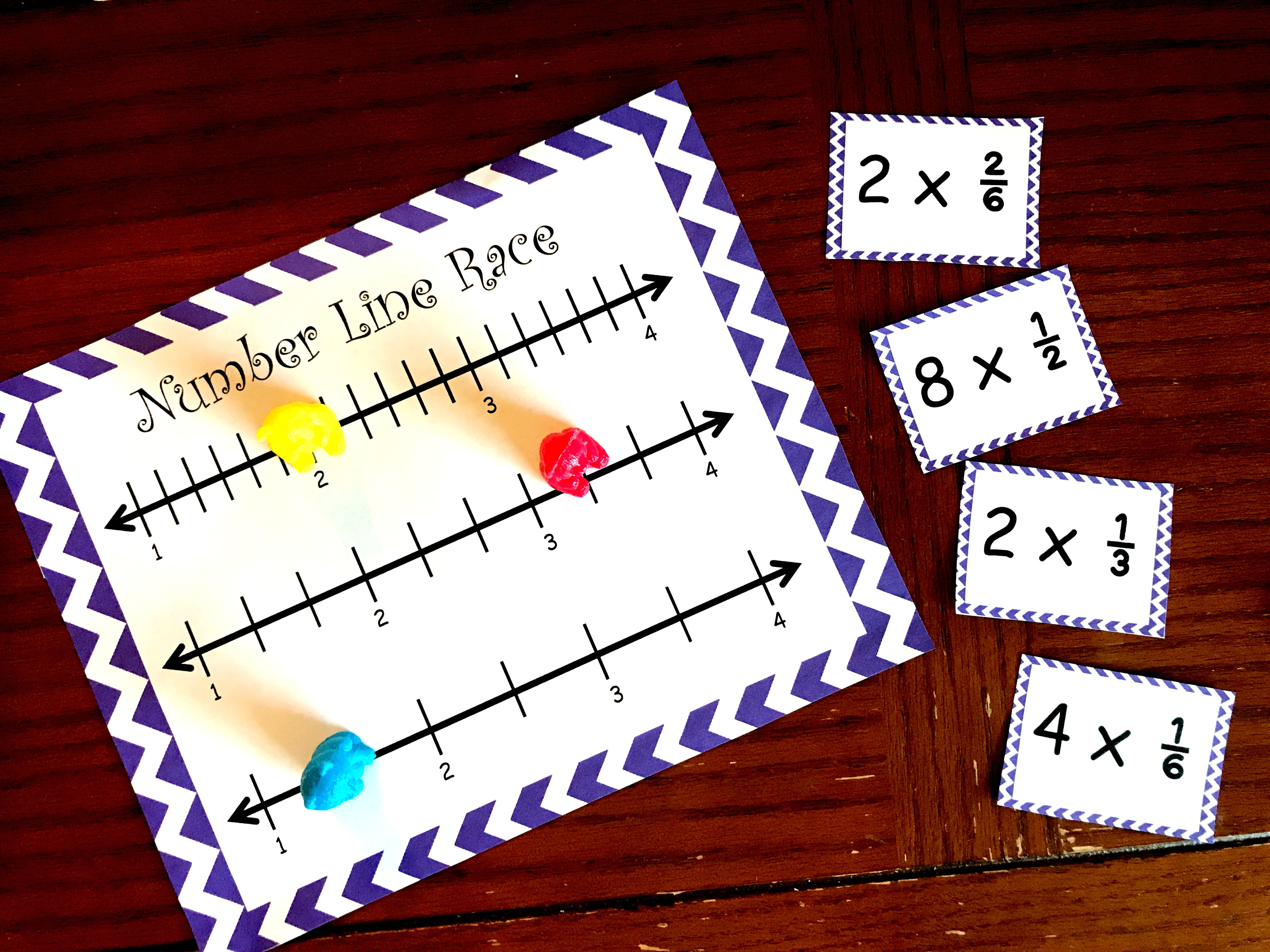Or this printable fraction game for simplifying fractions